# Pentagon

Within a regular pentagon ABCDE point, P is such that the triangle is equilateral ABP. How big is the angle BCP?
Make a sketch.

x =  66 °

### Step-by-step explanation: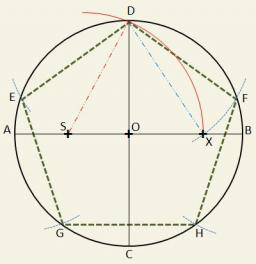Did you find an error or inaccuracy? Feel free to write us. Thank you!Tips to related online calculators

#### You need to know the following knowledge to solve this word math problem:

We encourage you to watch this tutorial video on this math problem:

## Related math problems and questions:

• PentagonCalculate the area of a regular pentagon, which diagonal is u=16.
• Equilateral triangle ABCIn the equilateral triangle ABC, K is the center of the AB side, the L point lies on one-third of the BC side near the point C, and the point M lies in the one-third of the side of the AC side closer to the point A. Find what part of the ABC triangle cont
• Candy - MOGretel deploys to the vertex of a regular octagon different numbers from one to eight candy. Peter can then choose which three piles of candy give Gretel others retain. The only requirement is that the three piles lie at the vertices of an isosceles trian
• PentagonCalculate the length of side, circumference and area of a regular pentagon, which is inscribed in a circle with radius r = 6 cm.
• Diagonal in rectangle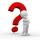In that rectangle ABCD is the center of BC point E and point F is center of CD. Prove that the lines AE and AF divide diagonal BD into three equal parts.
• Regular n-gonWhich regular polygon have a radius of circumscribed circle r = 10 cm and the radius of inscribed circle p = 9.962 cm?
• ConstructConstruct a triangle ABC inscribed circle has a radius r = 2 cm, the angle alpha = 50 degrees = 8 cm. Make a sketch, analysis, construction and description.
• Regular hexagonal pyramid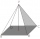Calculate the height of a regular hexagonal pyramid with a base edge of 5 cm and a wall height of w = 20cm. Sketch a picture.
• Inscribed and described circle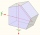Find the radii of a circle inscribed and circumscribed by a regular pentagon whose side measures 3 cm.
• Regular 5-gonCalculate the area of the regular pentagon with side 7 cm.
• Regular n-gon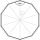In a regular n-angle polygon, the internal angle is 144 degrees. Find the number n indicating the number of sides of this polygon.
• Katy MO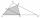Kate drew triangle ABC. The middle of the line segment AB has marked as X and the center of the side AC as Y. On the side BC wants to find the point Z such that the content area of a 4gon AXZY was greatest. What part of the triangle ABC can maximally occu
• Isosceles - isoscelesIt is given a triangle ABC with sides /AB/ = 3 cm /BC/ = 10 cm, and the angle ABC = 120°. Draw all points X such that true that BCX triangle is an isosceles and triangle ABX is isosceles with the base AB.
• Hexagon in circleCalculate the radius of a circle whose length is 10 cm greater than the circumference of a regular hexagon inscribed in this circle.
• Triangle anglesIn a triangle, ABC, the interior angle at vertex C is twice the internal angle at point A. The outer angle at point B measured 117 degrees. How big is the external angle at vertex A?
• Nine-gonCalculate the perimeter of a regular nonagon (9-gon) inscribed in a circle with a radius 13 cm.
• Hexagonal pyramidPlease calculate the height of a regular hexagonal pyramid with a base edge of 5cm and a wall height of w = 20cm. Please sketch a picture.